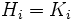# Isomorph-freeness is strongly join-closed

This article gives the statement, and possibly proof, of a subgroup property (i.e., isomorph-free subgroup) satisfying a subgroup metaproperty (i.e., strongly join-closed subgroup property)
View all subgroup metaproperty satisfactions | View all subgroup metaproperty dissatisfactions |Get help on looking up metaproperty (dis)satisfactions for subgroup properties
Get more facts about isomorph-free subgroup |Get facts that use property satisfaction of isomorph-free subgroup | Get facts that use property satisfaction of isomorph-free subgroup|Get more facts about strongly join-closed subgroup property

## Statement

### Verbal statement

Suppose$G$ is a group, and$H_i, i \in I$ is a collection of isomorph-free subgroups of$G$ for some (possibly empty) indexing set$I$. Then, the join of the$H_i$ is also an isomorph-free subgroup of$G$.

## Proof

Given: A group$G$, a collection$H_i, i \in I$ of isomorph-free subgroups of$G$ for some (possibly empty) indexing set$I$.

To prove: The join of the$H_i$s is also isomorph-free.

Proof: Suppose$H$ is the join of the$H_i$s, and suppose$K$ is a subgroup of$G$ isomorphic to$H$. Let$\alpha:H \to K$ be an isomorphism, and let$K_i = \alpha(H_i)$. Now, since$\alpha$ is an isomorphism,$H_i \cong K_i$ for each$i \in I$. By assumption,$H_i$ is isomorph-free in$G$, so$H_i = K_i$ for each$i \in I$. Thus, the join of the$H_i$s equals the join of the$K_i$s, forcing$H = K$.